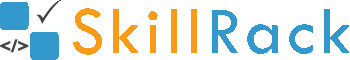Must Solve 100 Programs for Campus Placement Part - 8/104 months ago

1) Sum - Last Three Digits

The program must accept an integer N as the input. The program must print the sum of the last three digits of N as the output.

Boundary Condition(s):
100 <= N <= 10^8

Example Input/Output 1:
Input:
2019

Output:
10

Example Input/Output 2:
Input:
1546

Output:
15

Click the link below to solve this program
http://www.skillrack.com/solve/9225

2) Predecessor and Successor of X

The program must accept N integers and an integer X as the input. The program must print the (X-1)th integer. Then the program must print the Xth integer. Then the program must print the (X+1)th integer.

Boundary Condition(s):
3 <= N <= 100
1 < X < N

Example Input/Output 1:
Input:
7
12 45 78 56 89 23 10
4

Output:
78 56 89

Click the link below to solve this program
http://www.skillrack.com/solve/9224

3) Find the Element

The program must accept N integers and an integer M as the input. The program must print yes if M is present in the N integers. Else the program must print no as the output.

Boundary Condition(s):
1 <= N <= 100
1 <= Each integer value, M <= 10^5

Example Input/Output 1:
Input:
6
91 73 64 82 50 33
73

Output:
yes

Click the link below to solve this program
http://www.skillrack.com/solve/9222

4) String Reversal

The program must accept a string S as the input. The program must reverse the string S and print it as the output.

Boundary condition(s):
2 <= Length of S <= 100

Example Input/Output 1:
Input:
skillrack

Output:
kcarlliks

Example Input/Output 2:
Input:
technology

Output:
ygolonhcet

Click the link below to solve this program
http://www.skillrack.com/solve/9221

5) Consonants in First X Characters

The program must accept a string S and an integer X as the input. The program must print all the consonants in the first X characters of S as the output. If there is no consonant in the first X characters of S then the program must print -1 as the output.

Boundary Condition(s):
1 <= Length of S <= 100

Example Input/Output 1:
Input:
assurance
5

Output:
ssr

Example Input/Output 2:
Input:
aeiousky
4

Output:
-1

Click the link below to solve this program
http://www.skillrack.com/solve/9220

6) Previous and Next - Alphabets

The program must accept a lower case alphabet CH as the input. The program must print the previous alphabet and the next alphabet of CH as the output.
Note:
For the alphabet a, consider the previous alphabet as z.
For the alphabet z, consider the next alphabet as a.

Example Input/Output 1:
Input:
c

Output:
bd

Example Input/Output 2:
Input:
z

Output:
ya

Click the link below to solve this program
http://www.skillrack.com/solve/9219

7) Smallest and Largest - Sum

The program must accept three integers as the input. The program must print the sum of the smallest integer and the largest integer as the output.

Example Input/Output 1:
Input:
2 5 3

Output:
7

Example Input/Output 2:
Input:
0 1 3

Output:
3

Click the link below to solve this program
http://www.skillrack.com/solve/9218

8) Remove Special Characters

The program must accept a string S as the input. The program must remove all the special characters (other than the alphabets and digits) from the string S. Then the program must print the modified string S as the output.

Boundary Condition(s):
1 <= Length of S <= 100

Example Input/Output 1:
Input:
**skillrack#123***

Output:
skillrack123

Example Input/Output 2:
Input:
*ke@##123KEy

Output:
ke123KEy

Click the link below to solve this program
http://www.skillrack.com/solve/9217

9) Smallest to Largest Integer

Accept two integers X and Y as the input and print all the integers from the smallest integer to the largest integer as the output.

Example Input/Output 1:
Input:
10 5

Output:
5 6 7 8 9 10

Example Input/Output 2:
Input:
7 10

Output:
7 8 9 10

Click the link below to solve this program
http://www.skillrack.com/solve/9216

10) Print All Vowels

Accept a string S as the input and print all the vowels in S as the output. If there is no vowel in S then the program must print -1 as the output.

Boundary Condition(s):
1 <= Length of S <= 100

Example Input/Output 1:
Input:
SkillRack

Output:
ia

Example Input/Output 2:
Input:
Sky

Output:
-1

Click the link below to solve this program
http://www.skillrack.com/solve/9215